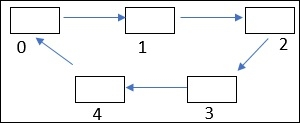# C++ Program to Check Cycle in a Graph using Topological Sort

C++Server Side ProgrammingProgramming

#### C in Depth: The Complete C Programming Guide for Beginners

45 Lectures 4.5 hours

#### Practical C++: Learn C++ Basics Step by Step

Most Popular

50 Lectures 4.5 hours

#### Master C and Embedded C Programming- Learn as you go

66 Lectures 5.5 hours

In a Directed Acyclic Graph, we can sort vertices in linear order using topological sort.

Topological sort is only work on Directed Acyclic Graph. In a Directed Acyclic Graph (DAG), there can be more than one topological sort.

We shall consider a C++ program, which will perform topological sort to check cycle in a graph.

## For example## Algorithms

Topological Sort:
Begin
Declare topo_sort(int *v, int T_S[], int i) function
a = new NodeInfo.
a->n = i
a->S_Time = cn.
Call push_node(a) function to insert data.
v[i] = 1.
for (int j = 0; j < 5; j++)
if (T_S[i][j] == 0 || (T_S[i][j] == 1 && v[j] == 1)) then
continue.
else if(T_S[i][j] == 1 && v[j] == 0) then
cn++.
Call topo_sort(v,T_S, j) function.
cn++.
a = pop().
a->L_Time = cn.
Store_Node(a).
End.

## Example

#include<iostream>
#include<conio.h>
using namespace std;
struct NodeInfo {
int n;
int L_Time, S_Time;
}
*a = NULL;
struct Node {
NodeInfo *ptr;
Node *nxt;
}
*t = NULL, *b = NULL, *npt = NULL;
NodeInfo *ptr1;
}
*hd = NULL, *m = NULL, *n = NULL, *npt1 = NULL;
int cn = 0;
bool flag = false;
void push_node(NodeInfo *pt) { //insert data
npt = new Node;
npt->ptr = pt;
npt->nxt = NULL;
if (t == NULL) {
t = npt;
} else {
npt->nxt = t;
t = npt;
}
}
NodeInfo *pop() {
if (t == NULL) {
cout<<"underflow\n";
} else {
b = t;
t = t->nxt;
return(b->ptr);
delete(b);
}
}
void Store_Node(NodeInfo *pt1) { //store data
npt1 = new Node_Link;
npt1->ptr1 = pt1;
npt1->lk = NULL;
if (cn == 0) {
hd = npt1;
m = hd;
m->lk = NULL;
cn++;
} else {
m = hd;
npt1->lk = m;
hd = npt1;
}
}
void delete_node(int x) { //delete node
m = hd;
if ((m->ptr1)->n == x) {
hd = hd->lk;
delete(m);
} else {
while ((m->ptr1)->n != x && m->lk != NULL) {
n = m;
m = m->lk;
}
if ((m->ptr1)->n == x) {
n->lk = m->lk;
delete(m);
} else if (m->lk == NULL) {
flag = true;
cout<<"There is no circle in this graph\n";
}
}
}
void topo_sort(int *v, int T_S[], int i) { //performing topological sort
a = new NodeInfo;
a->n = i;
a->S_Time = cn;
push_node(a);
v[i] = 1;
for (int j = 0; j < 5; j++) {
if (T_S[i][j] == 0 || (T_S[i][j] == 1 && v[j] == 1))
continue;
else if(T_S[i][j] == 1 && v[j] == 0) {
cn++;
topo_sort(v,T_S,j);
}
}
cn++;
a = pop();
a->L_Time = cn;
Store_Node(a);
return;
}
void topologic_sort(int *v, int T_S[], int i) {
v[i] = 1;
delete_node(i);
for (int j = 0; j < 5; j++) {
if (T_S[i][j] == 0 || (T_S[i][j] == 1 && v[j] == 1)) {
continue;
} else if(T_S[i][j] == 1 && v[j] == 0) {
topologic_sort(v, T_S, j);
}
}
return;
}
void Insert_Edge(int T_S[], int source, int destination) { // insert the value of edge.
T_S[source][destination] = 1;
return;
}
int main() {
int v, T_S, T_S_N, cn = 0, a, b;
for (int i = 0; i < 5; i++) {
v[i] = 0;
}
for (int i = 0; i < 5; i++) {
for (int j = 0; j < 5; j++) {
T_S[i][j] = 0;
}
}
while (cn < 5) {
cout<<"Enter the source: ";
cin>>a;
cout<<"Enter the destination: ";
cin>>b;
cout<<endl;
Insert_Edge(T_S, a, b);
cn++;
}
topo_sort(v, T_S, 0);
for (int i = 0; i < 5; i++) {
v[i] = 0;
for (int j = 0; j < 5; j++) {
T_S_N[j][i] = T_S[i][j];
}
}
if (hd != NULL) {
topologic_sort(v, T_S_N, (hd->ptr1)->n);
if (flag == false) {
cout<<"There is a cycle in this graph...\n";
}
}
getch();
}

## Output

Enter the source: 0
Enter the destination: 1

Enter the source: 1
Enter the destination: 2

Enter the source: 2
Enter the destination: 3

Enter the source: 3
Enter the destination: 4

Enter the source: 4
Enter the destination: 0

There is a cycle in this graph...